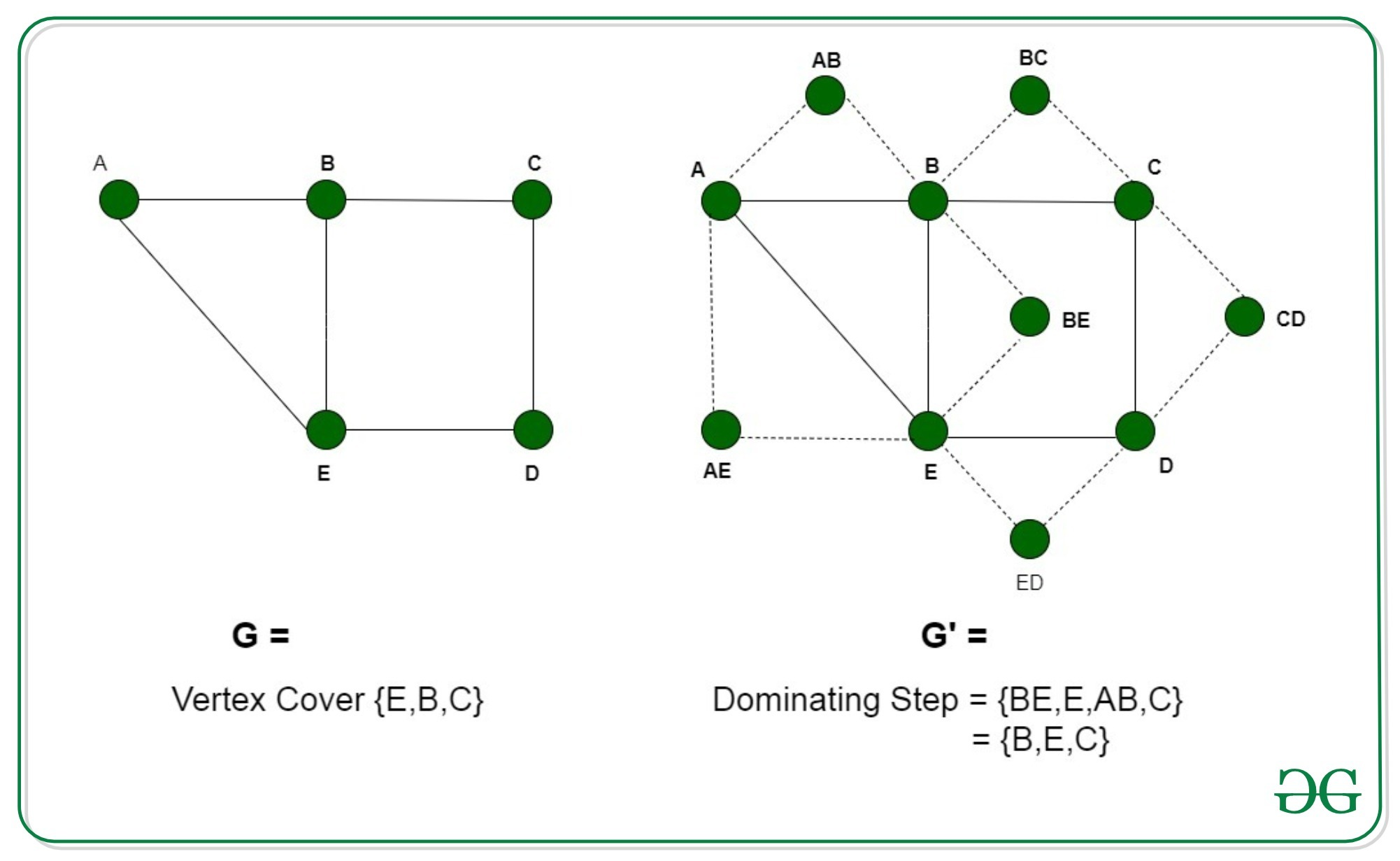Open in App
Not now

# Proof that Dominant Set of a Graph is NP-Complete

• Difficulty Level : Expert
• Last Updated : 05 Dec, 2022

Pre-requisite: Dominant Set of a Graph, NP-Complete

A dominating set in a graph G = (V, E) is a subset of vertices V’ following the condition that the vertices not belonging in V’ are adjacent to some vertex in V’.

Problem: Given a graph G(V, E) and an integer k, the problem is to determine if the graph has a Dominating Set of size k. Explanation: An instance of the problem is an input specified to the problem. An instance of the Dominating Set problem is a graph G (V, E) and an integer k, and the problem is to check whether the graph can have a dominating set in G. Since an NP-Complete problem, by definition, is a problem which is both in NP and NP-hard, the proof for the statement that a problem is NP-Complete consists of two parts:

1. Dominating Set is NP Complete If any problem is in NP, then, given a ‘certificate’, which is a solution to the problem and an instance of the problem (a graph G and a positive integer k, in this case), we will be able to verify (check whether the solution given is correct or not) the certificate in polynomial time. The certificate is a sequence of vertices forming a Dominating Set in the graph. We can validate this solution by checking that all the vertices belong to the vertices of the graph and all the vertices that are not a part of this sequence are adjacent to some of the vertex in this set. This can be done in polynomial time, that is O(V +E) using the following strategy :
```flag=true
for every vertex v in V:
if v doesn't belong to Dominating Set:
verify the set of edges
corresponding to v
to any of the vertices in DS,
set flag=False and break
if (flag)
solution is correct
else
solution is incorrect```
1. Dominating Set is NP-Hard In order to prove that the Dominating Set is NP-Hard, we will have to reduce a known NP-Hard problem to this problem. We will carry out a reduction from the Vertex Cover problem to the Dominating Set problem. Every instance of the Vertex Cover problem consists of a graph G = (V, E) and an integer k consisting of the subset of vertices as the input can be converted to a Dominating Set problem consisting of graph G’ = (V’, E’). We will construct the graph G’ in the following way:
• E’ = For every edge E consisting of vertices {u, v} in the graph G, add a new vertex {uv} and individually join it to the new vertices u and v.
• V’ = Add all the vertices V of the original graph G.
• Let us assume that the graph G has a vertex cover VC of size k. Every edge in G has one of the vertices belonging to the vertex cover. Therefore, for every edge e, consisting of vertices {u, v} at least either u or v is a part of the vertex cover. So, if u is contained in VC, then the adjacent vertex is v, is also covered by some of the elements in VC. Now, for all the newly added vertices UV for each edge, the vertex is adjacent to both u and v, one of which is at least a part of the VC, as proved above. Therefore, the additional vertices for all the edges are also covered by this VC. The set of vertices forming the Vertex Cover of size k form Dominating Set in graph G’. Therefore, if G has a vertex cover G’ has a dominating set of the same size.
• We assume that the graph G’ has a Dominating Set of size k. Two possibilities may arise, either the vertex in the DS is an original vertex or it belongs to the newly added vertex UV for each edge {u, v}. In the second case, since every new vertex is connected to the two vertices of the edge, u and v, therefore, it can be replaced by either u or v. Since, these three vertices form a triangle, therefore, even by substituting view with either u or v, we can continue to span all the vertices that were spanned before replacing. This will lead to the elimination of all the newly added vertices while spanning all the edges of the graph G’. The newly added vertices are dominated by the modified DS and cover all the edges in G with at least either u or v for each edge UV. Therefore, if G’ has a Dominating Set of size k, G will have a vertex cover with size utmost k .

In the following figure, the vertex B dominates both AB and BE, therefore, it can be easily replaced. Therefore, these two vertices are redundant.Thus we can say that the graph G’ contains a dominating set if graph G contains vertex cover. Therefore, any instance of the dominating set problem can be reduced to an instance of the vertex cover problem. Thus, the dominating set is also NP-Hard. Since vertex cover is in both NP and NP-Hard classes, the dominant Set of a Graph is NP-Complete.

My Personal Notes arrow_drop_up
Related Articles